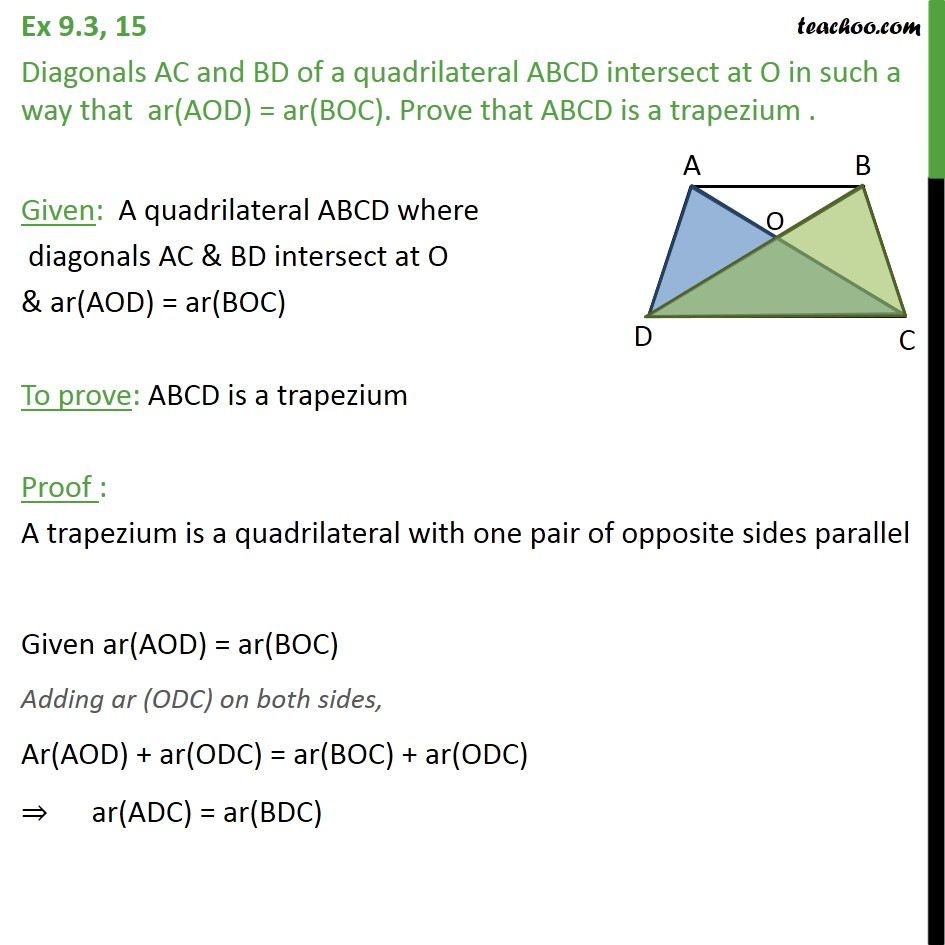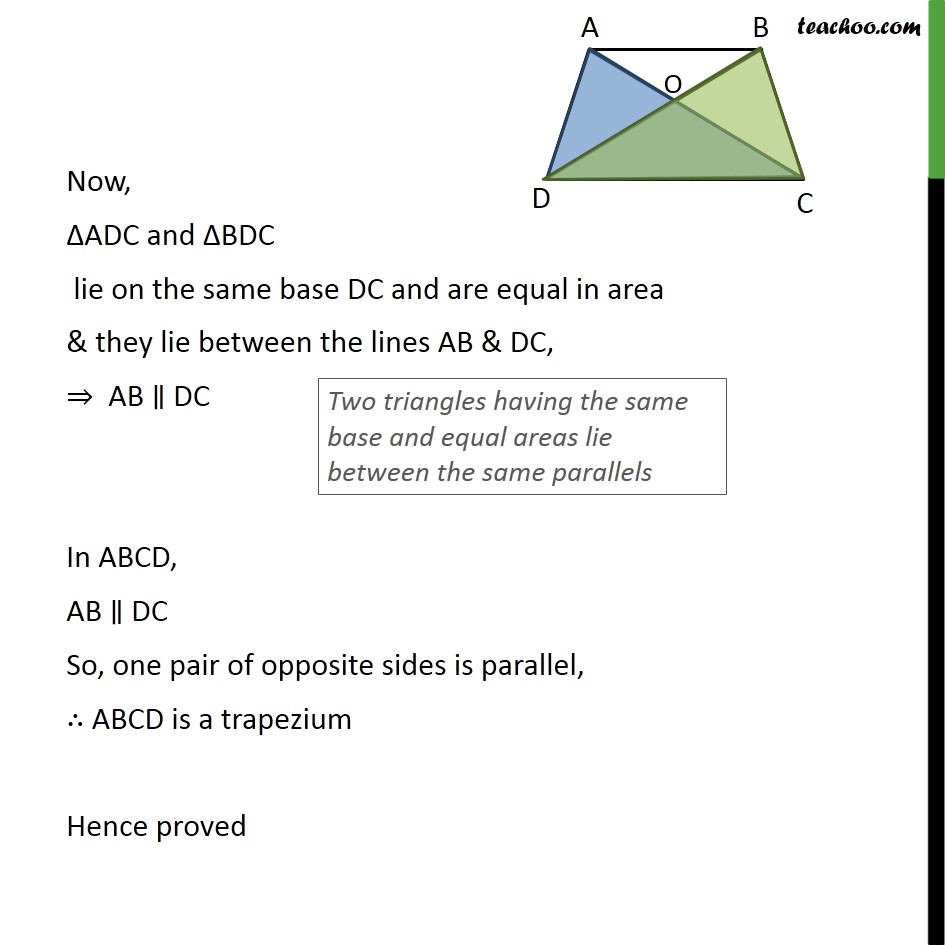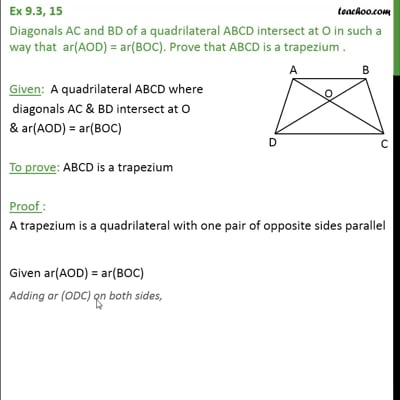Ex 9.3

Chapter 9 Class 9 - Areas of Parallelograms and Triangles [Deleted]
Serial order wiseThis video is only available for Teachoo black users

Solve all your doubts with Teachoo Black (new monthly pack available now!)

### Transcript

Ex 9.3, 15 Diagonals AC and BD of a quadrilateral ABCD intersect at O in such a way that ar(AOD) = ar(BOC). Prove that ABCD is a trapezium . Given: A quadrilateral ABCD where diagonals AC & BD intersect at O & ar(AOD) = ar(BOC) To prove: ABCD is a trapezium Proof : A trapezium is a quadrilateral with one pair of opposite sides parallel Given ar(AOD) = ar(BOC) Adding ar (ODC) on both sides, Ar(AOD) + ar(ODC) = ar(BOC) + ar(ODC) ⇒ ar(ADC) = ar(BDC) Now, ΔADC and ΔBDC lie on the same base DC and are equal in area & they lie between the lines AB & DC, ⇒ AB ∥ DC In ABCD, AB ∥ DC So, one pair of opposite sides is parallel, ∴ ABCD is a trapezium Hence proved